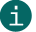# Check digit calculation of Modulo 16

Modulo 16

## This is a description of calculating check digits according to Modulo 16.ActiveBarcode calculates this check digits for most codes automatically.
This documentation is only for the sake of completeness.

Calculation of a checksum according to Modulo 16:
Modulo 16 is used by the barcode symbology Codabar. Here is an example of how to calculate the check digit according to Modulo 16:

 Digits: A 7 8 9 A Reference numbers: 16 7 8 9 16 Sum of reference numbers: 56 Calculate checksum: 56 / 16 = 3 Remainder 8 difference: 16 - 8 = 8 Check digit Reference number 8 = 8

Reference numbers:
 00 = 0 01 = 1 02 = 2 03 = 3 04 = 4 05 = 5 06 = 6 07 = 7 08 = 8 09 = 9 10 = - 11 = \$ 12 = : 13 = / 14 = . 15 = + 16 = A 17 = B 18 = C 19 = D